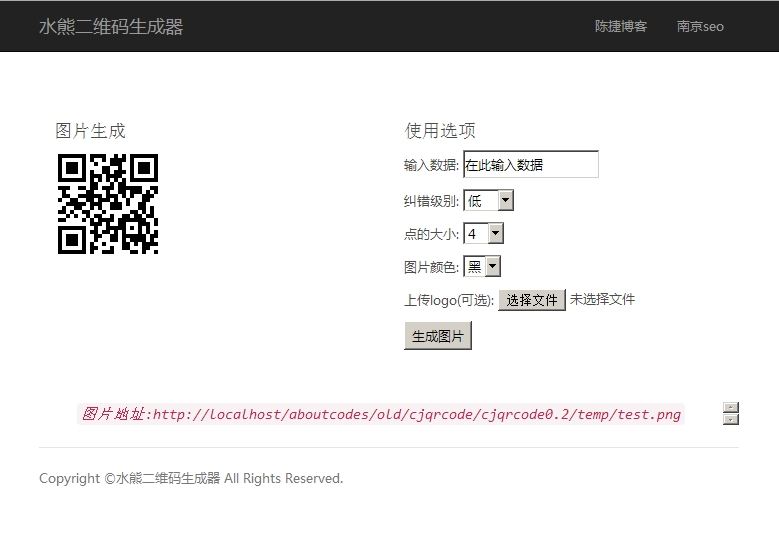# 使用php实现二维码生成并结合使用bootstrap响应式布局

文件输出二维码
$data = '测试数据'; // 生成的文件名$filename = $errorCorrectionLevel.'|'.$matrixPointSize.'.png';
// 纠错级别：L、M、Q、H
$errorCorrectionLevel = 'L'; // 点的大小：1到10$matrixPointSize = 4;
QRcode::png($data,$filename, $errorCorrectionLevel,$matrixPointSize, 2);

，一个背景色：
$col = ImageColorAllocate($base_image,255,255,255);
$col = ImageColorAllocate($base_image,0,0,0);

$QR = imagecreatefromstring(file_get_contents($QR));
$logo = imagecreatefromstring(file_get_contents($logo));
$QR_width = imagesx($QR);
$QR_height = imagesy($QR);
$logo_width = imagesx($logo);
$logo_height = imagesy($logo);
$logo_qr_width =$QR_width / 4;
$scale =$logo_width / $logo_qr_width;$logo_qr_height = $logo_height /$scale;
$from_width = ($QR_width - $logo_qr_width) / 2; imagecopyresampled($QR, $logo,$from_width, $from_width, 0, 0,$logo_qr_width, $logo_qr_height,$logo_width, $logo_height); //修改文件名，和无logo的文件名做区分，因为logo不同，所以文件名加入mt_rand随机数 imagepng($QR,\$filename);## 《使用php实现二维码生成并结合使用bootstrap响应式布局》有3个想法

1.一支穿云箭说道：

下载http://1drv.ms/1hj9h1C 哥，这个下载连接用不了了，能不能发这个二维码的代码给我学习一下，万分感谢
邮箱spybbb@163.com

1.chenjie说道：
2.一支穿云箭说道：

感谢陈哥 💡